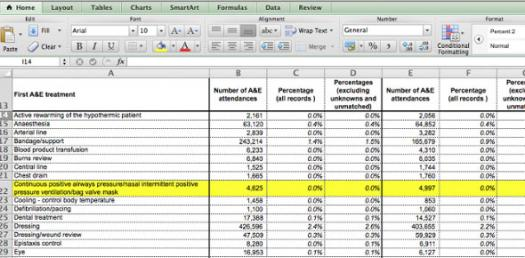# Microsoft Excel - Level 1

10 Questions | Attempts: 1927
ShareSettingsIf you feel confident with using MS Excel at Level 1 - Try the quiz and test your knowledge. If not, please return to the tutorials for further support.

• 1.
What is the correct equation you would insert in the cell to solve the following:  2 is what percent of 8?
• A.

(1/8)-100

• B.

(2/8)*100

• C.

(3/12)*100

• D.

(2*8)/100

• 2.
Determine what percentage 6 is of 24 by using the following equation 6/24*100?
• A.

30%

• B.

24%

• C.

25%

• D.

6%

• 3.
What are you trying to achieve by using the following symbol %?
• A.

Create a total?

• B.

Deduct from equation?

• C.

Total up the sum?

• D.

Calculate a percentage?

• 4.
Which do you press to enter the current time in a cell?
• A.

CTRL+SHIFT+: (colon)

• B.

CTRL+; (semicolon)

• C.

CTRL+F10

• 5.
Which Excel feature can summarise the data in a large table?
• A.

Data Validation

• B.

Conditonal Formatting

• C.

Autoformat

• D.

Pivot Table

• 6.
Which option would you select to alter the formatting properties in a cell?
• A.

Format Cells

• B.

Pick from List

• C.

Insert

• D.

Clear contents

• 7.
Which of the following things can be entered in a cell?
• A.

Numbers

• B.

Words

• C.

Equations, Formulas or Functions

• D.

Fill Colour

• 8.
Which formula can add all the numeric values in a range of cells, ignoring those that are not numeric, and place the result in a different cell?
• A.

Count

• B.

Average

• C.

Sum

• 9.
To select a column the easiest method is to...?
• A.

Double click any cell in the column

• B.

Drag from the top cell in the column to the last cell in the column

• C.

• D.

Click the column label

• 10.
If you press _____, the cell accepts your typing as its contents but the focus stays in the cell
• A.

ENTER

• B.

CTRL + ENTER

• C.

TAB

• D.

INSERT

## Related TopicsBack to top
×

Wait!
Here's an interesting quiz for you.